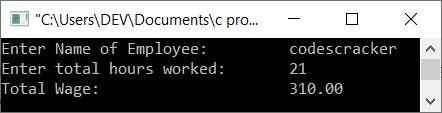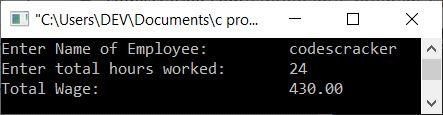# C program to calculate the wage of labor on a daily basis

In this post, we will learn how to create a program in C that will calculate and print the total daily wage of labor. Wage means the salary or money that has to be paid to the worker. Labor is the man who worked for you. The money that has to be paid to the labor will be paid on a daily basis as per the following wage structure:

Hours Worked Rate Applicable
Until the first eight hours 50.00
For the next four hours 10.00 per hour additional
For the next four hours 20.00 per hour additional
For the next four hours 25.00 per hour additional
For the next four hours 40.00 per hour additional

## Find and Print Labor Wages in C

The question is: write a program in C to compute the wage of labor working on a daily basis as per the wage structure provided in the above table. And the result should be as follows:

```Enter Name of Employee:      XXXXXXXX
Enter total hours worked:    21
Total Wage:                  310```

The program given below is the answer to the above question:

```#include<stdio.h>
#include<conio.h>
int main()
{
float initWage=50, hours, tempHour, tempWage, totalWage;
char name;
printf("Enter Name of Employee: \t");
gets(name);
printf("Enter total hours worked: \t");
scanf("%f", &hours);
if(hours<=8)
totalWage = initWage;
else if(hours>8 && hours<=12)
{
tempHour = hours-8;
tempWage = tempHour*10;
totalWage = tempWage + initWage;
}
else if(hours>12 && hours<=16)
{
tempHour = hours-12;
tempWage = 4*10;
totalWage = initWage + tempWage + (tempHour*20);
}
else if(hours>16 && hours<=20)
{
tempHour = hours-16;
tempWage = (4*10) + (4*20);
totalWage = initWage + tempWage + (tempHour*25);
}
else if(hours>20 && hours<=24)
{
tempHour = hours-20;
tempWage = (4*10) + (4*20) + (4*25);
totalWage = initWage + tempWage + (tempHour*40);
}
else
{
printf("A single day only has 24 hours.");
getch();
return 0;
}
printf("Total Wage: \t\t\t%0.2f", totalWage);
getch();
return 0;
}```

As the program was written in the Code::Blocks IDE, here is the sample run after a successful build and run:Now provide the name of the laborer, say codescracker, and the total number of hours worked by him, say 21. Press the ENTER key to see the wage of labor as shown in the second snapshot of the sample run given here:Here is another sample run. In this case, the laborer has worked for all the day (24 hours). Let's see what the labor earnings will be for one full day based on the above pay scale:Here are some of the main steps used in the above program:

• Ask the user to enter his name.
• Again, ask him to enter how many hours he has worked for any particular date (or for today).
• Never forget to initialize a variable, say initWage with 50 as its initial value at the start of the program. Because according to the pre-defined wage structure given above, the employer has to pay \$50 to the employee for his first eight hours of work. No matter whether he has worked only for 1 hours or a total of 8 hours.
• Now check whether the hour worked is less than or equal to 8 or not.
• If it is, then just initialize the value of initWage to the variable totalWage, which will hold up the total wage of the labor at the end of the program.
• If the worked hour is not less than or equal to eight, determine whether it is greater than eight and less than or equal to twelve.
• If it is, then add the rate given for the next 4 hours after the initial 8 hours worked by the labor, i.e., 10 rupees per hour is the extra rate for the next 4 hours after starting with 8 hours, which means we have to minus the given hour by 8 and then multiply the remaining hour value with 10, and then add it up with 50 to initialize it to the totalWage of labor.
• In this way, continue checking and calculating for the given number of hours.
• For example, if labor has worked 23 hours in a day, then the wage that has to be paid to labor will be calculated as follows:
• Because the user specified 23 as the total number of hours worked, 23 will be set as the hours variable.
• As 23 is greater than 20 and less than or equal to 24, therefore, the program flow goes inside the last else if block where the applied condition is:
`else if(hours>20 && hours<=24)`
As the rate for the first 8 hours is 50 rupees, the rate for the next 4 hours is \$10/hour additional, the rate for the next 4 hours is \$20/hour additional, the rate for the next 4 hours is \$25/hour additional, and the rate for the next and last 4 hours is \$40/hour additional.
• As a result, we must initialize the variable totalWage with 50 + (4*10) + (4*20) + (4*25) + ((hours-20)*40), 50 + 80 + 100 + ((23-20) *40), 230 + (3*40), 230 + 120, or 350. totalWage will hold the total wage in this program.
• Finally, print the value of totalWage as output.

C Quiz

« Previous Program Next Program »